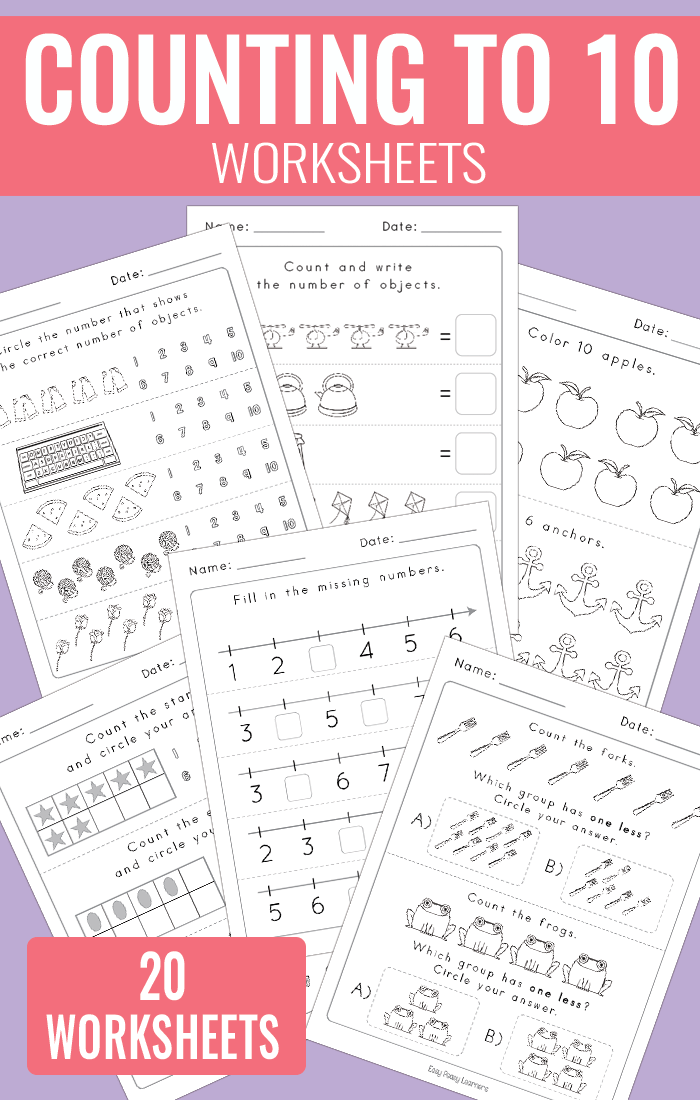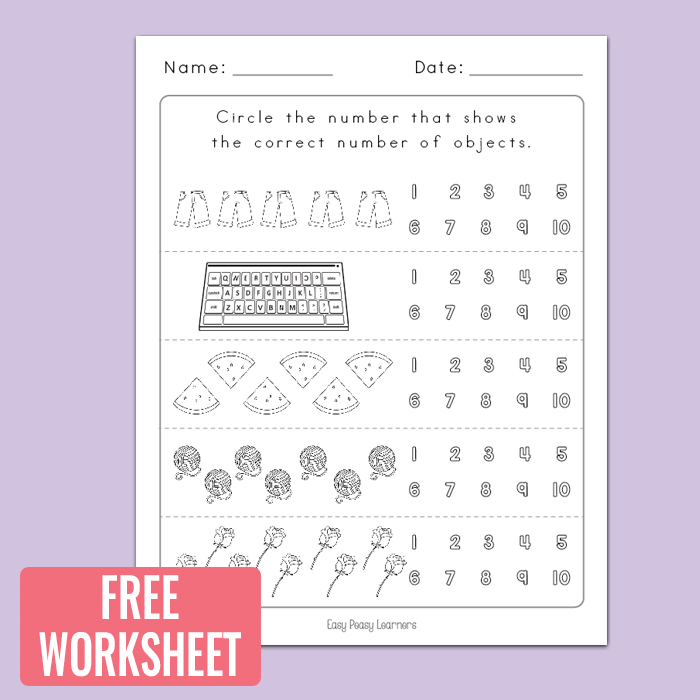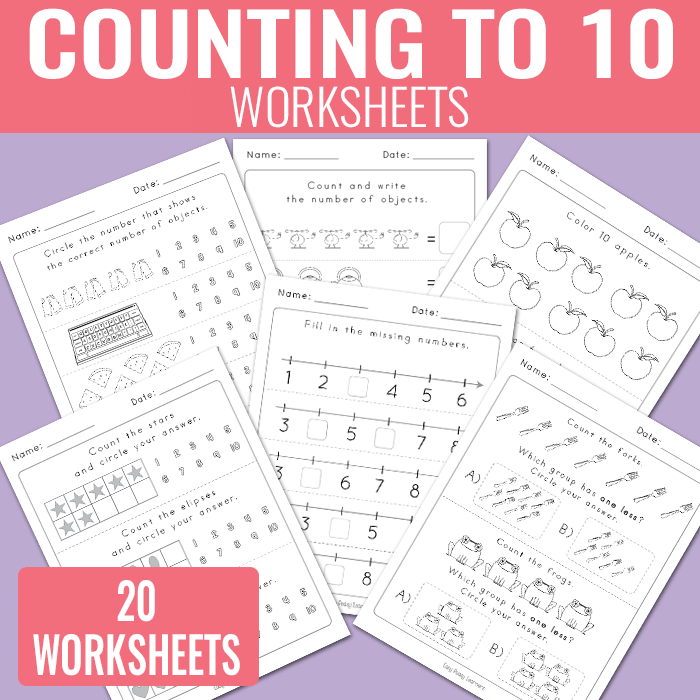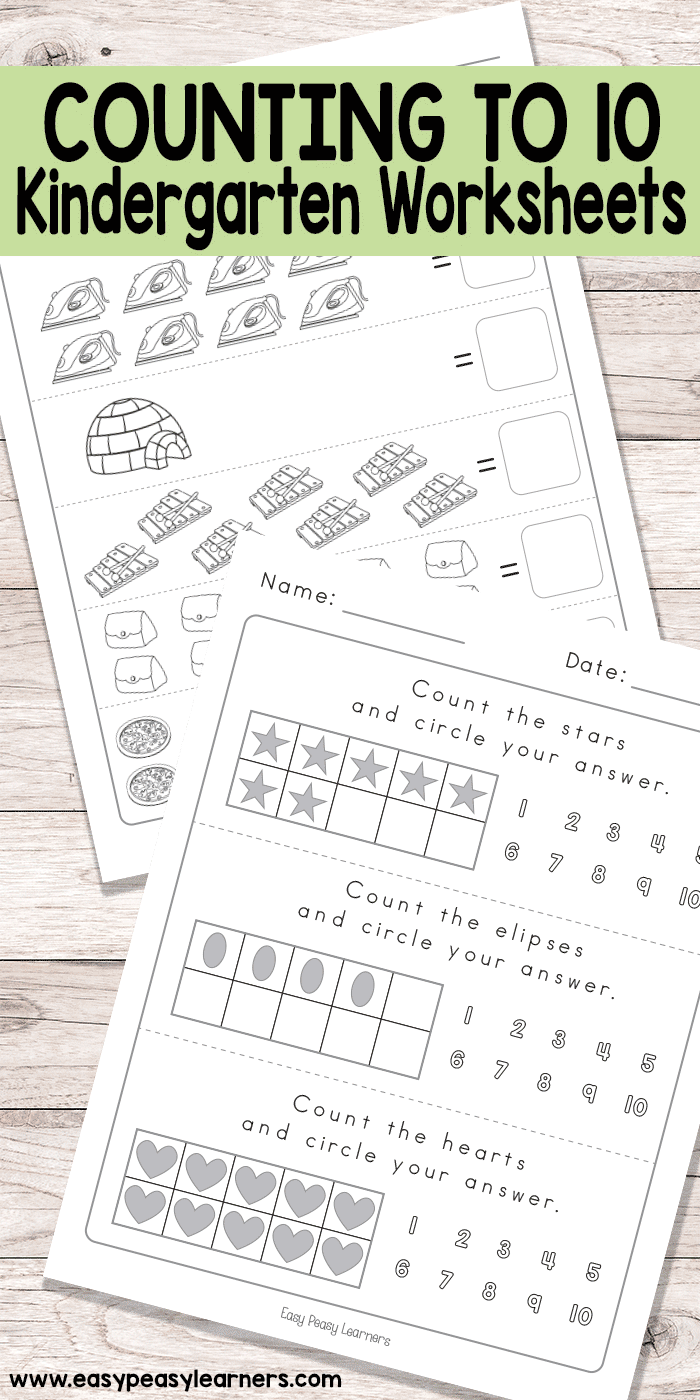# Counting to 10 Worksheets – Kindergarten Math Worksheets

If you are done with counting to 10, grab these counting to 10 worksheets and let’s continue counting.## Counting to 10 Worksheets – Kindergarten

This printable pack contains 20 unique worksheets

What to expect from this worksheets set;

• 2 worksheets ask the students to count the objects and circle the correct number.
• 1 worksheet where students have to count and match the object to the number by drawing a line.
• 2 worksheets where students have to count and write the number of objects
• 2 worksheets where students are asked to count the objects in ten frames and circle their answers.
• 5 where students are asked to color a specific number of objects.
• 4 worksheets feature ten frames where students are asked to count the shapes within the ten frame and circle their answers.
• 2 worksheets with numbered lines where students are asked to fill in the missing numbers.
• 4 worksheets where students are asked to circle the group that has one more or one less than the original group.

You can view the whole set at Teachers Pay Teachers where you can also preview the whole set.

Get Count to 10 Worksheets

Get Count to 10 Worksheets

These worksheets can be laminated for continuous use.Get Count to 10 Worksheets

Get Count to 10 Worksheets

More Kindergarten Worksheets for Kids

Counting to 3 Worksheets for Kindergarten

Counting to 5 Worksheets

Fill in the Missing Letters Worksheets

Dot-to-Dot Alphabet Worksheets

Picture Crossword Puzzles – perfect for spelling